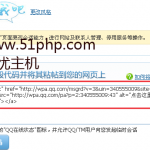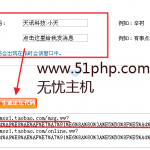# 【佛山云主机】如何给WORDPRESS主题添加独立的控制选项`1` `
 `2` `\$themename` `= ``"XXX主题"``;    ``//主题名称`
 `3` `\$shortname` `= ``"jiuchuangzhuti"``;    ``//主题简写，必须是英文、数字、下划线组合`
 `4` `\$options` `= ``array` `(`
 `5` `array``(``"name"` `=> ``"这里是大标题"``,``"type"` `=> ``"heading"``,``"desc"` `=> ``"这里是描述信息"``),`
 `6` `array``(``"name"` `=> ``"这里是小标题"``,``"id"` `=> ``\$shortname``.``"_test"``,``"std"` `=> ``"这里是默认值"``,``"type"` `=> ``"text"``)`
 `7` `);`
 `8` `function` `mytheme_add_admin() {`
 `9` `global` `\$themename``, ``\$shortname``, ``\$options``;`
 `10` `if` `( ``\$_GET``[``'page'``] == ``basename``(``__FILE__``) ) {`
 `11` `if` `( ``'save'` `== ``\$_REQUEST``[``'action'``] ) {`
 `12` `foreach` `(``\$options` `as` `\$value``) {`
 `13` `update_option( ``\$value``[``'id'``], ``\$_REQUEST``[ ``\$value``[``'id'``] ] ); }`
 `14` `foreach` `(``\$options` `as` `\$value``) {`
 `15` `if``( isset( ``\$_REQUEST``[ ``\$value``[``'id'``] ] ) ) { update_option( ``\$value``[``'id'``], ``\$_REQUEST``[ ``\$value``[``'id'``] ]  ); } ``else` `{ delete_option( ``\$value``[``'id'``] ); } }`
 `16` `//这里的 settings.php 就是这个文件的名称`
 `17` `header(``"Location: themes.php?page=settings.php&saved=true"``);`
 `18` `die``;`
 `19` `} ``else` `if``( ``'reset'` `== ``\$_REQUEST``[``'action'``] ) {`
 `20` `foreach` `(``\$options` `as` `\$value``) {`
 `21` `delete_option( ``\$value``[``'id'``] );`
 `22` `update_option( ``\$value``[``'id'``], ``\$value``[``'std'``] );`
 `23` `}`
 `24` `header(``"Location: themes.php?page=settings.php&reset=true"``);    ``//这里的 settings.php 就是这个文件的名称`
 `25` `die``;`
 `26` `}`
 `27` `}`
 `28` `add_theme_page(``\$themename``.``" Options"``, ``"\$themename 设置"``, ``'edit_themes'``, ``basename``(``__FILE__``), ``'mytheme_admin'``);`
 `29` `}`
 `30` `function` `mytheme_admin() {`
 `31` `global` `\$themename``, ``\$shortname``, ``\$options``;`
 `32` `if` `( ``\$_REQUEST``[``'saved'``] ) ``echo` `'

'``.``\$themename``.``' 设置已保存。

'``;`
 `33` `if` `( ``\$_REQUEST``[``'reset'``] ) ``echo` `'

'``.``\$themename``.``' 设置已重置。

'``;`
 `34` `?>`
 `35` `
 `36` `th{text-align:left;}`
 `37` `input{width:100%;}`
 `38` `.submit{width:100px;padding:0;}`
 `39` `.defaultbutton{padding-left:745px;}`
 `40` ``
 `41` `
`
 `42` `

`
 `43` `
`
 `44` `
`
 `45` ``
 `46` ``
 `47` ``
 `48` ``
 `49` `
 `50` `if` `(``\$value``[``'type'``] == ``"text"``) { ?>`
 `51` `
`
 `52` `
:
`
 `53` `
`
 `54` `"` `id=``""` `type=``""` `value=``""` `size=``"40"` `/>`
 `55` ``
 `56` ``
 `57` ``
 `58` `
`
 `59` `

`
 `60` `

`
 `61` `

`
 `62` ``
 `63` ``
 `64` `
 `65` `}`
 `66` `?>`
 `67` ``
 `68` `
`
 `69` `
`
 `70` ``
 `71` ``
 `72` ``
 `73` ``
 `74` `
`
 `75` `
`
 `76` ``
 `77` ``
 `78` ``
 `79` ``
 `80` ``
 `81` `
 `82` `}`
 `83` `add_action(``'admin_menu'``, ``'mytheme_add_admin'``);`
 `84` `?>`1 开通云主机、域名  2 建设官方网站 3 整体网站推广 … 成功见证 创业技能素质培训# 【简单云】WORDPRESS主题如何添加QQ和旺旺在线聊天窗口`1` `<``a` `target``=``"_blank"` `href``=``"复制的代码到这里 alt="``这里可以任意填写备注0"`
 `2` `src``=``"http://img04.taobaocdn.com/tps/i4/T1uUG.XjtkXXcb2gzo-77-19.gif"`
 `3` `id``=``"1"``type``=``"1"``>`1 开通云主机、域名  2 建设官方网站 3 整体网站推广 … 成功见证 创业技能素质培训